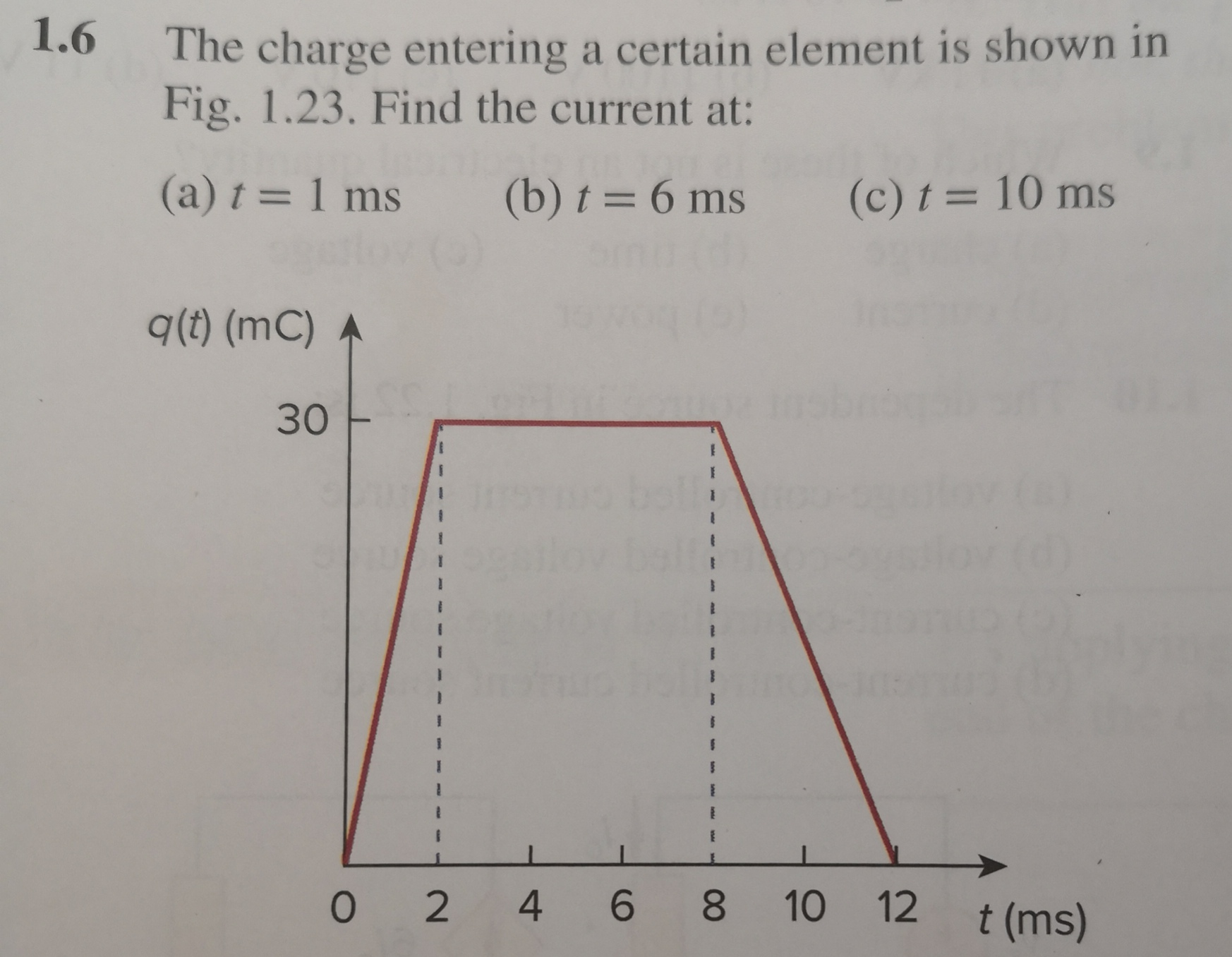# 1.6 The charge entering a certain element is shown in Fig. 1.23. Find the current at: (a) t = 1 ms (b) t = 6 ms q(t) (mC) (c) t = 10 ms 30 O 2 4 6 8 10 12 t (ms)

Question

At t=1ms is the i=12(a estimated number)/1=12A or is it i=30/2=15A.help_outlineImage Transcriptionclose1.6 The charge entering a certain element is shown in Fig. 1.23. Find the current at: (a) t = 1 ms (b) t = 6 ms q(t) (mC) (c) t = 10 ms 30 O 2 4 6 8 10 12 t (ms) fullscreen

### Want to see the step-by-step answer?